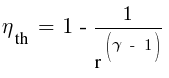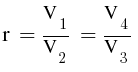# Otto Cycle problem

• PauloBuzon

#### PauloBuzon

1. Homework Statement

An Otto engine has a clearance volume of 7%. It produces 300 kW of power. What is the amount of heat rejected in kW?

## Homework Equations

(v2=Vc)Clearance Volume = (c)Clearance% x (Vd)Volume Displacement (v1-v2)where r is the compression ratio## The Attempt at a Solution

rk(compression ratio) = (c+1)/c stuck i don't know where to start
then i should be able to solve the problem where Qrejected = Qadded - Work

Last edited:

Your missing an equation for efficiency.

Your missing an equation for efficiency.
i just edited it

Energy balance:
$$\dot{W} = \dot{Q}_{in} - \dot{Q}_{out}$$
Efficiency definition:
$$n_{th} = \frac{\dot{W}}{\dot{Q}_{in}}$$
Otto cycle efficiency:
$$n_{th} = 1- \frac{1}{r^{(\gamma-1)}}$$
Compression ratio definition:
$$r = \frac{V_d + V_{cc}}{V_{cc}}$$
You get 4 equations, 4 unknowns (##\dot{Q}_{in}##, ##\dot{Q}_{out}##, ##n_{th}##, ##r##), so you can resolve them. ##V_d## and ##V_{cc}## are not known but their ratio is given in the problem (and it is all that is really needed):
$$0.07 = \frac{V_{cc}}{V_d}$$
(or it might be ##0.07 = \frac{V_{cc}}{V_d + V_{cc}}##; Not clear from the statement -> 7% of what?)

Energy balance:
$$\dot{W} = \dot{Q}_{in} - \dot{Q}_{out}$$
Efficiency definition:
$$n_{th} = \frac{\dot{W}}{\dot{Q}_{in}}$$
Otto cycle efficiency:
$$n_{th} = 1- \frac{1}{r^{(\gamma-1)}}$$
Compression ratio definition:
$$r = \frac{V_d + V_{cc}}{V_{cc}}$$
You get 4 equations, 4 unknowns (##\dot{Q}_{in}##, ##\dot{Q}_{out}##, ##n_{th}##, ##r##), so you can resolve them. ##V_d## and ##V_{cc}## are not known but their ratio is given in the problem (and it is all that is really needed):
$$0.07 = \frac{V_{cc}}{V_d}$$
(or it might be ##0.07 = \frac{V_{cc}}{V_d + V_{cc}}##; Not clear from the statement -> 7% of what?)
7% clearance volume , clearance volume = cVd = c(v1-v2) so 0.07 = c(v1-v2)